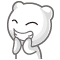# 博客

## UOJ Easy Round #2 题解

2015-05-17 17:47:23 By vfleaking

### 手机的生产

from wangyisong1996

#### 算法三

UER的A题当然是水水的啦！### 信息的交换

from jiry_2

#### 算法三

\begin{align*} f_{i,j}=\sum_{k=i+1}^j [A_i>A_k] \times f_{k,j} \times f_{i+1,k-1} \end{align*}

\begin{align*} f_{i,j}=\sum_{k=i+1}^j [A_i>A_k] \times f_{k,j} \times f_{i+1,k-1} \times 2^{h_{i,k-1}} \end{align*}

### 谣言的传播

from Glaceon08，题目是 vfleaking 造的。

#### 算法三

1. 如果 $i$ 和 $j$ 在同一个内向树内，且 $j$ 是 $i$ 的祖先，那么影响系数是 $f_i - f_j + 1$。
2. 如果 $i$ 和 $j$ 在同一个基环内向树内（即看成无向图后，在同一个连通块内），$j$ 在环上，设环长为 $l$ 以及 $i$ 所在内向树的根到 $j$ 的距离为 $s$，那么影响系数就是 $f_i - l + s + 1$。
3. 其它情况答案均为 $f_i$。

#### 算法四

• 如果 $j$ 不在环上且是 $i$ 的祖先那么对答案贡献 $-f_j + 1$
• 如果 $j$ 在环上且和 $i$ 在同一个基环内向树那么对答案贡献 $-l + s + 1$ （符号见前文）

## 评论

matthew99

wangyisong1996

osu

Tunix

Rating_Jia_Jia

Gromah

Rating_Jia_Jia

Arksandom

shiyukun

zhouyuheng

myee
T3 最小解也可以直接构造。 先对树上节点取其父亲，如果不行就跳过；再对环上节点取其父亲，如果不行就跳过；剩下的节点两两匹配一下就好了。 这是一份实现：https://uoj.ac/submission/622607。